Paul's Online Notes
Home / Algebra / Solving Equations and Inequalities / Linear Inequalities
Show Mobile Notice Show All Notes Hide All Notes
Mobile Notice
You appear to be on a device with a "narrow" screen width (i.e. you are probably on a mobile phone). Due to the nature of the mathematics on this site it is best views in landscape mode. If your device is not in landscape mode many of the equations will run off the side of your device (should be able to scroll to see them) and some of the menu items will be cut off due to the narrow screen width.

### Section 2.11 : Linear Inequalities

To this point in this chapter we’ve concentrated on solving equations. It is now time to switch gears a little and start thinking about solving inequalities. Before we get into solving inequalities we should go over a couple of the basics first.

At this stage of your mathematical career it is assumed that you know that

$a < b$

means that $$a$$ is some number that is strictly less than $$b$$. It is also assumed that you know that

$a \ge b$

means that $$a$$ is some number that is either strictly bigger than $$b$$ or is exactly equal to $$b$$. Likewise, it is assumed that you know how to deal with the remaining two inequalities. > (greater than) and $$\le$$ (less than or equal to).

What we want to discuss is some notational issues and some subtleties that sometimes get students when they really start working with inequalities.

First, remember that when we say that $$a$$ is less than $$b$$ we mean that $$a$$ is to the left of $$b$$ on a number line. So,

$- 1000 < 0$

is a true inequality.

Next, don’t forget how to correctly interpret $$\le$$ and $$\ge$$. Both of the following are true inequalities.

$4 \le 4\hspace{0.25in}\hspace{0.25in}\hspace{0.25in} - 6 \le 4$

In the first case 4 is equal to 4 and so it is “less than or equal” to 4. In the second case -6 is strictly less than 4 and so it is “less than or equal” to 4. The most common mistake is to decide that the first inequality is not a true inequality. Also be careful to not take this interpretation and translate it to < and/or >. For instance,

$4 < 4$

is not a true inequality since 4 is equal to 4 and not strictly less than 4.

Finally, we will be seeing many double inequalities throughout this section and later sections so we can’t forget about those. The following is a double inequality.

$- 9 < 5 \le 6$

In a double inequality we are saying that both inequalities must be simultaneously true. In this case 5 is definitely greater than -9 and at the same time is less than or equal to 6. Therefore, this double inequality is a true inequality.

On the other hand,

$10 \le 5 < 20$

is not a true inequality. While it is true that 5 is less than 20 (so the second inequality is true) it is not true that 5 is greater than or equal to 10 (so the first inequality is not true). If even one of the inequalities in a double inequality is not true then the whole inequality is not true. This point is more important than you might realize at this point. In a later section we will run across situations where many students try to combine two inequalities into a double inequality that simply can’t be combined, so be careful.

The next topic that we need to discuss is the idea of interval notation. Interval notation is some very nice shorthand for inequalities and will be used extensively in the next few sections of this chapter.

The best way to define interval notation is the following table. There are three columns to the table. Each row contains an inequality, a graph representing the inequality and finally the interval notation for the given inequality.

Inequality Graph Interval Notation
$a \le x \le b$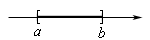$\left[ {a,b} \right]$
$a < x < b$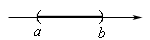$\left( {a,b} \right)$
$a \le x < b$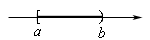$\left[ {a,b} \right)$
$a < x \le b$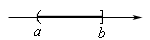$\left( {a,b} \right]$
$x > a$$\left( {a,\infty } \right)$
$x \ge a$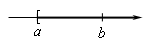$\left[ {a,\infty } \right)$
$x < b$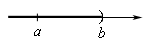$\left( { - \infty ,b} \right)$
$x \le b$$\left( { - \infty ,b} \right]$

Remember that a bracket, “[” or “]”, means that we include the endpoint while a parenthesis, “(” or “)”, means we don’t include the endpoint.

Now, with the first four inequalities in the table the interval notation is really nothing more than the graph without the number line on it. With the final four inequalities the interval notation is almost the graph, except we need to add in an appropriate infinity to make sure we get the correct portion of the number line. Also note that infinities NEVER get a bracket. They only get a parenthesis.

We need to give one final note on interval notation before moving on to solving inequalities. Always remember that when we are writing down an interval notation for an inequality that the number on the left must be the smaller of the two.

It’s now time to start thinking about solving linear inequalities. We will use the following set of facts in our solving of inequalities. Note that the facts are given for <. We can however, write down an equivalent set of facts for the remaining three inequalities.

1. If $$a < b$$ then $$a + c < b + c$$ and $$a - c < b - c$$for any number $$c$$. In other words, we can add or subtract a number to both sides of the inequality and we don’t change the inequality itself.

2. If $$a < b$$ and $$c > 0$$ then $$ac < bc$$ and $$\frac{a}{c} < \frac{b}{c}$$. So, provided $$c$$ is a positive number we can multiply or divide both sides of an inequality by the number without changing the inequality.

3. If $$a < b$$ and $$c < 0$$ then $$ac > bc$$ and $$\frac{a}{c} > \frac{b}{c}$$. In this case, unlike the previous fact, if $$c$$ is negative we need to flip the direction of the inequality when we multiply or divide both sides by the inequality by $$c$$.

These are nearly the same facts that we used to solve linear equations. The only real exception is the third fact. This is the important fact as it is often the most misused and/or forgotten fact in solving inequalities.

If you aren’t sure that you believe that the sign of $$c$$ matters for the second and third fact consider the following number example.

$- 3 < 5$

I hope that we would all agree that this is a true inequality. Now multiply both sides by 2 and by -2.

\begin{align*}- 3 & < 5\hspace{0.5in} & - 3 & < 5\\ - 3\left( 2 \right) & < 5\left( 2 \right)\hspace{0.5in} & - 3\left( { - 2} \right) & > 5\left( { - 2} \right)\\ - 6 & < 10 \hspace{0.5in} & 6 & > - 10\end{align*}

Sure enough, when multiplying by a positive number the direction of the inequality remains the same, however when multiplying by a negative number the direction of the inequality does change.

Okay, let’s solve some inequalities. We will start off with inequalities that only have a single inequality in them. In other words, we’ll hold off on solving double inequalities for the next set of examples.

The thing that we’ve got to remember here is that we’re asking to determine all the values of the variable that we can substitute into the inequality and get a true inequality. This means that our solutions will, in most cases, be inequalities themselves.

Example 1 Solving the following inequalities. Give both inequality and interval notation forms of the solution.
1. $$- 2\left( {m - 3} \right) < 5\left( {m + 1} \right) - 12$$
2. $$2\left( {1 - x} \right) + 5 \le 3\left( {2x - 1} \right)$$
Show All Solutions Hide All Solutions
Show Discussion

Solving single linear inequalities follow pretty much the same process for solving linear equations. We will simplify both sides, get all the terms with the variable on one side and the numbers on the other side, and then multiply/divide both sides by the coefficient of the variable to get the solution. The one thing that you’ve got to remember is that if you multiply/divide by a negative number then switch the direction of the inequality.

a $$- 2\left( {m - 3} \right) < 5\left( {m + 1} \right) - 12$$ Show Solution

There really isn’t much to do here other than follow the process outlined above.

\begin{align*} - 2\left( {m - 3} \right) & < 5\left( {m + 1} \right) - 12\\ - 2m + 6 & < 5m + 5 - 12\\ - 7m & < - 13\\ m & > \frac{{13}}{7}\end{align*}

You did catch the fact that the direction of the inequality changed here didn’t you? We divided by a “-7” and so we had to change the direction. The inequality form of the solution is $$m > \frac{{13}}{7}$$. The interval notation for this solution is, $$\left( {\frac{{13}}{7},\infty } \right)$$.

b $$2\left( {1 - x} \right) + 5 \le 3\left( {2x - 1} \right)$$ Show Solution

Again, not much to do here.

\begin{align*}2\left( {1 - x} \right) + 5 & \le 3\left( {2x - 1} \right)\\ 2 - 2x + 5 & \le 6x - 3\\ 10 & \le 8x\\ \frac{{10}}{8} & \le x\\ \frac{5}{4} & \le x\end{align*}

Now, with this inequality we ended up with the variable on the right side when it more traditionally on the left side. So, let’s switch things around to get the variable onto the left side. Note however, that we’re going to need also switch the direction of the inequality to make sure that we don’t change the answer. So, here is the inequality notation for the inequality.

$x \ge \frac{5}{4}$

The interval notation for the solution is $$\left[ {\frac{5}{4},\infty } \right)$$.

Now, let’s solve some double inequalities. The process here is similar in some ways to solving single inequalities and yet very different in other ways. Since there are two inequalities there isn’t any way to get the variables on “one side” of the inequality and the numbers on the other. It is easier to see how these work if we do an example or two so let’s do that.

Example 2 Solve each of the following inequalities. Give both inequality and interval notation forms for the solution.
1. $$- 6 \le 2\left( {x - 5} \right) < 7$$
2. $$- 3 < \frac{3}{2}\left( {2 - x} \right) \le 5$$
3. $$- 14 < - 7\left( {3x + 2} \right) < 1$$
Show All Solutions Hide All Solutions
a $$- 6 \le 2\left( {x - 5} \right) < 7$$ Show Solution

The process here is fairly similar to the process for single inequalities, but we will first need to be careful in a couple of places. Our first step in this case will be to clear any parenthesis in the middle term.

$- 6 \le 2x - 10 < 7$

Now, we want the $$x$$ all by itself in the middle term and only numbers in the two outer terms. To do this we will add/subtract/multiply/divide as needed. The only thing that we need to remember here is that if we do something to middle term we need to do the same thing to BOTH of the out terms. One of the more common mistakes at this point is to add something, for example, to the middle and only add it to one of the two sides.

Okay, we’ll add 10 to all three parts and then divide all three parts by two.

$\begin{array}{c} 4 \le 2x < 17\\ 2 \le x < \displaystyle \frac{{17}}{2}\end{array}$

That is the inequality form of the answer. The interval notation form of the answer is $$\left[ {2,\frac{{17}}{2}} \right)$$.

b $$- 3 < \frac{3}{2}\left( {2 - x} \right) \le 5$$ Show Solution

In this case the first thing that we need to do is clear fractions out by multiplying all three parts by 2. We will then proceed as we did in the first part.

$\begin{array}{c} - 6 < 3\left( {2 - x} \right) \le 10\\ - 6 < 6 - 3x \le 10\\ - 12 < - 3x \le 4\end{array}$

Now, we’re not quite done here, but we need to be very careful with the next step. In this step we need to divide all three parts by -3. However, recall that whenever we divide both sides of an inequality by a negative number we need to switch the direction of the inequality. For us, this means that both of the inequalities will need to switch direction here.

$4 > x \ge - \frac{4}{3}$

So, there is the inequality form of the solution. We will need to be careful with the interval notation for the solution. First, the interval notation is NOT $$\left( {4, - \frac{4}{3}} \right]$$. Remember that in interval notation the smaller number must always go on the left side! Therefore, the correct interval notation for the solution is $$\left[ { - \frac{4}{3},4} \right)$$.

Note as well that this does match up with the inequality form of the solution as well. The inequality is telling us that $$x$$ is any number between 4 and $$- \frac{4}{3}$$ or possibly $$- \frac{4}{3}$$ itself and this is exactly what the interval notation is telling us.

Also, the inequality could be flipped around to get the smaller number on the left if we’d like to. Here is that form,

$- \frac{4}{3} \le x < 4$

When doing this make sure to correctly deal with the inequalities as well.

c $$- 14 < - 7\left( {3x + 2} \right) < 1$$ Show Solution

Not much to this one. We’ll proceed as we’ve done the previous two.

$\begin{array}{c} - 14 < - 21x - 14 < 1\\ 0 < - 21x < 15\end{array}$

Don’t get excited about the fact that one of the sides is now zero. This isn’t a problem. Again, as with the last part, we’ll be dividing by a negative number and so don’t forget to switch the direction of the inequalities.

$\begin{array}{c} \displaystyle 0 > x > - \frac{{15}}{{21}}\\ \displaystyle 0 > x > - \frac{5}{7}\hspace{0.25in}{\mbox{OR}}\hspace{0.25in} - \frac{5}{7} < x < 0\end{array}$

Either of the inequalities in the second row will work for the solution. The interval notation of the solution is $$\left( { - \frac{5}{7},0} \right)$$.

When solving double inequalities make sure to pay attention to the inequalities that are in the original problem. One of the more common mistakes here is to start with a problem in which one of the inequalities is < or > and the other is $$\le$$ or $$\ge$$, as we had in the first two parts of the previous example, and then by the final answer they are both < or > or they are both $$\le$$ or $$\ge$$. In other words, it is easy to all of a sudden make both of the inequalities the same. Be careful with this.

There is one final example that we want to work here.

Example 3 If $$- 1 < x < 4$$ then determine $$a$$ and $$b$$ in $$a < 2x + 3 < b$$.
Show Solution

This is easier than it may appear at first. All we are really going to do is start with the given inequality and then manipulate the middle term to look like the second inequality. Again, we’ll need to remember that whatever we do to the middle term we’ll also need to do to the two outer terms.

So, first we’ll multiply everything by 2.

$- 2 < 2x < 8$

$1 < 2x + 3 < 11$
We’ve now got the middle term identical to the second inequality in the problems statement and so all we need to do is pick off $$a$$ and $$b$$. From this inequality we can see that $$a = 1$$ and $$b = 11$$.